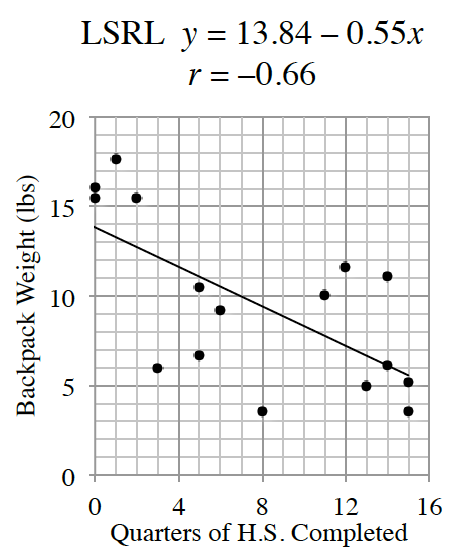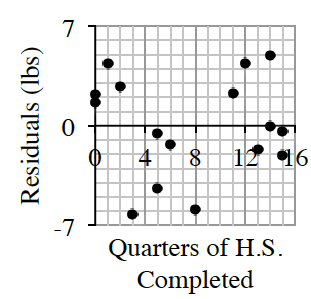### Home > GB8I > Chapter 7 Unit 8 > Lesson INT1: 7.1.3 > Problem7-40

7-40.
 Do the freshmen really have the largest backpacks, or is that just high school legend? Delenn was able to weigh a random sample of student backpacks throughout the school year. She also recorded the number of quarters of high school completed by the student who owned the backpack. Using spreadsheet software, Delenn created the following data representations:Interpret the slope of the $LSRL$ in the context of this study.Hint (a):What are the units associated with the slope? Is the slope positive or negative?Answer (a):On average, student backpacks get $0.55$ lbs lighter with each quarter of H.S. completed.Calculate and interpret $R$-squared in context.Hint (b):Square $r$ to find $R$-squared.Answer (b):_______ % of the variability in ______________ can be explained by a linear relationship with _______________.What is the residual with the greatest magnitude and what point does it belong to?Hint (c):Which point is farthest from the $LSRL$, or which pointis farthest from the $x$-axis on the residual plot?Answer (c):The largest residual is 6.2 lbs, relating to a student who has completed $3$ quarters of H.S.Using the $LSRL$ model, estimate the weight of a backpack for a student who has completed $10$ quarters of high school. Use appropriate precision in your answer.Hint (d):Let $x = 10$ in the given $LSRL$ equation.Is a linear model the best choice for predicting backpack weight in this study? Support your answer.Hint (e):Does the residual plot have random scatter?

Quarters Completed

Backpack Weight (lbs)

$0$

$16.03$

$0$

$15.47$

$8$

$3.52$

$12$

$11.62$

$1$

$17.66$

$5$

$6.67$

$5$

$10.48$

$13$

$4.96$

$2$

$15.47$

$6$

$9.21$

$14$

$6.10$

$14$

$11.10$

$3$

$5.96$

$11$

$10.06$

$15$

$3.54$

$15$

$5.18$